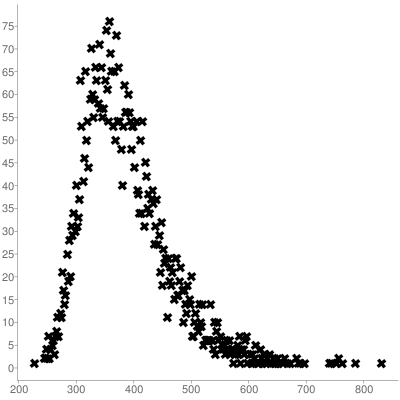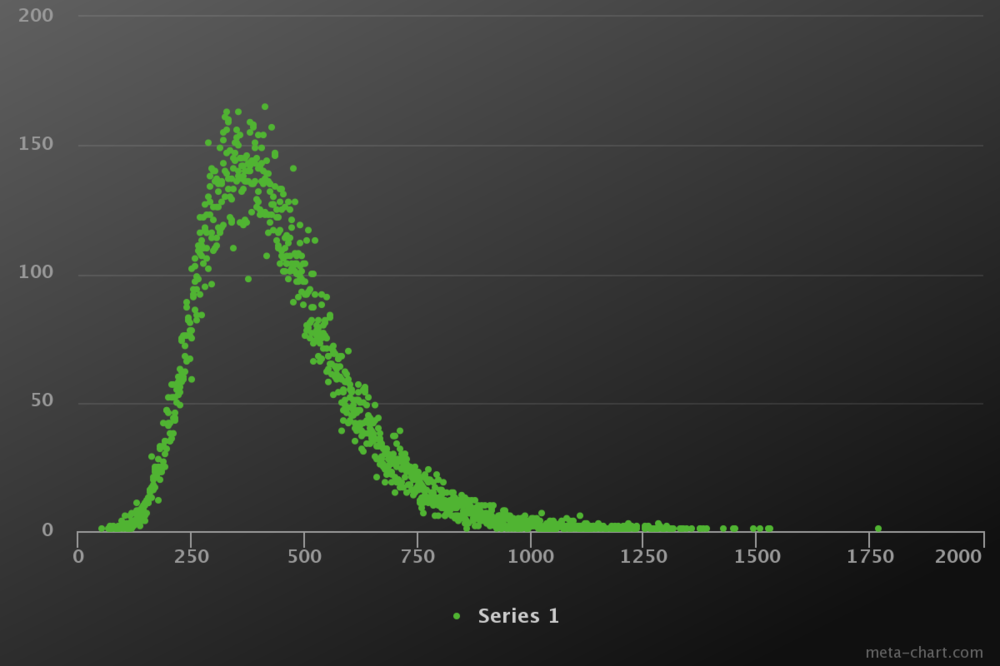Recommended Posts

I might make this more organized and add some more algorithms if I find a need to. I originally made this after a distribution that resembles reaction times and so it'd probably be useful after conditional waits and stuff as a simulation of reaction time...but generally also more believable for clicking. This is what the distribution looks like after generating ~5000 clicks with random between 200 and 1000:Oh, and it's also important to note that if you are going to use this, you'd need a larger random span than normal, for example 200-1000, because as you can see in the graph the chance is already very low at the center.

Code V.1

import java.util.Random;

/**
* Generates randoms by using a generalized extreme value distribution
*
* @author Reveance
*
*/
public class GEVRandom {

private Random random = null;

public GEVRandom() {
this(new Random());
}

public GEVRandom(Random random) {
this.setRandom(random);
}

/**
* Attempts to make a proper distribution of randoms where the function
* returns the numbers at the tails approximately 0 times when run for
* ~100000 times.
*
* @param min
* @param max
* @return A random value between min and max based on the distribution
*/
public int between(int min, int max) {
int span = (max - min);

int rand;
do {
double val = getRandomDouble((span / 3) - (span / 7), span / 14) + min;
rand = (int) Math.round(val);
} while (rand < min || rand > max);
return rand;
}

public double getRandomDouble(double location, double scale) {
double u = getRandom().nextDouble();
double x = getQuantile(u, location, scale);

return x;
}

public double getQuantile(double p, double a, double b) {
return a - b * Math.log(-Math.log(p));
}

public Random getRandom() {
return random;
}

public void setRandom(Random random) {
this.random = random;
}
}

I updated the class, this is actually the real Generalized extreme value distribution, which also allows for changing the shape (e.g. putting the top to the end etc.). This is what it looks like after 50000 calls to between(0, 1800):And here's the updated code:

import java.util.Random;

/**
* Generates randoms by using a generalized extreme value distribution
*
* @author Reveance
*
*/
public class GEVDRandom {

private Random random = null;

public GEVDRandom() {
this(new Random());
}

public GEVDRandom(Random random) {
this.setRandom(random);
}

/**
* Attempts to make a proper distribution of randoms where the function
* returns the numbers at the tails approximately 0 times when run for
* ~100000 times.
*
* @param min
* @param max
* @return A random value between min and max based on the distribution
*/
public int between(int min, int max) {
int span = (max - min);

int rand;
do {
double val = getRandomDouble(0 + span / 7, span / 14, -0.00000001);
rand = (int) Math.round(val);
} while (rand < min || rand > max);
return rand;
}

public double getRandomDouble(double location, double scale, double shape) {
double u = getRandom().nextDouble();
double x = getQuantile(u, location, scale, shape);

return x;
}

public double getQuantile(double p, double u, double o, double e) {
double log1dp = Math.log10(1 / p);

if (e == 0) {
return u + o * Math.log10(1 / log1dp);
} else {
return u + o * (Math.pow(log1dp, -e) - 1) / e;
}
}

public Random getRandom() {
return random;
}

public void setRandom(Random random) {
this.random = random;
}
}

Edited by Reveance
Code update
•2

Join the conversation

You can post now and register later. If you have an account, sign in now to post with your account.×   Pasted as rich text.   Paste as plain text instead

Only 75 emoji are allowed.

×   Your previous content has been restored.   Clear editor

×   You cannot paste images directly. Upload or insert images from URL.